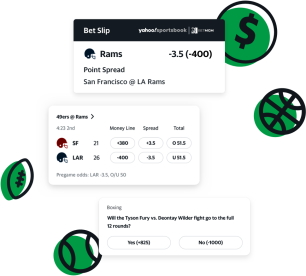It can be difficult, curious and intimidating to read the odds during sports betting for the first time. But understanding the probability and few secrets which can be used to read odds can make your job simple. It would not be a recommended move to bet on sports without knowing about the odds. This is the reason why it is important to read odds in sports betting. So, please continue scrolling to know more about how to read odds in sports betting.

## Learn about the types of odds

There are three famous types of odds which are fractional, decimal and money line. You have to be clear that there is not much difference to read and calculate sports odds between these three types. Thus, it will not affect your pay-out. These are just the three important types of odds through which a probability of an even can be calculated.## The fractional odds

A fractional odd can be defined as the ratio of profit to the initial bet. For example, if the ratio of a sports team is calculated using probability to be 9-1, then the meaning is that you will receive the amount at stake along with the nine times profit. Therefore, the potential return, whether a gain or loss for your stake amount, can be calculated as the value of your stake multiplied by the ratio. Let’s look at a small math example. If the stake amount is 10 and the ratio is 9/1, then the return would be [10 x (9/1)]. This is the main reason why simple math can play an effective role in reading odds and help you as odds work in betting.

##The decimal magic

The favorites and winning teams can be easily identified through the pay-out. There is no necessity of backing up the stakes in decimal odds as the total return would be calculated as a stake multiplied by an odd decimal number. One has to understand that the higher is the decimal odds, the lesser is the probability for the team in a sport to win.

## The signed money-line odds

Usually, plus (+) indicates positive, and a minus (-) indicates negative. But the one most important thing to remember while calculating odd through money-line is that minus indicates the favorites whereas plus indicates the unfavorable. Thus, before entering the betting world, it is important to learn to read odds and make your game tricky by involving some statistics to create favorably.